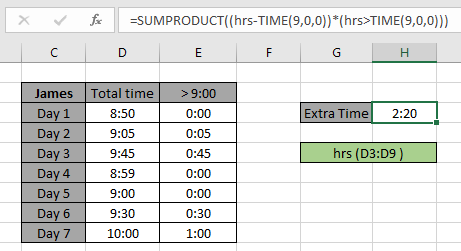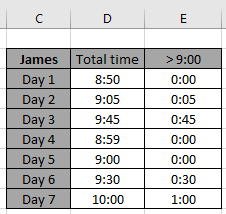How to Sum the Number of Hours an Employee Worked in ExcelIn this article, we will learn How to Sum the Number of Hours an Employee Worked in Excel.

Scenario

In simple words, while working with time data tables, sometimes we need to calculate time values from a table based on a condition. Condition is to get the sum of time values which are above 9 hours.

SUMPRODUCT formula in Excel

For this article we will be needing the use the following functions:

Now we will make a formula out of these functions. Here we will given the time value data and we needed sum time values where value is greater than 9 hours.

formula:

 = SUMPRODUCT ( ( times - TIME (9,0,0) ) * ( times > TIME (9,0,0) ) )

variables:

times: array of Time values

Example :

All of these might be confusing to understand. Let's understand how to use the function using an example. James has to complete 9 hrs daily but he gets to use the extra time above 9 hrs in the next week.

We have the time record of him for the last 7 days. And for recalculation purpose, we mentioned the extra time for each day.Here hrs ( D3:D9 ) given in as using named range.

Now we will use the following formula to get the SUM of time for the Month1 in F3 cell.

Use the Formula:

 = SUMPRODUCT ( ( hrs - TIME (9,0,0) ) * ( hrs > TIME (9,0,0) ) )

Explanation:

• TIME (9,0,0) returns the 9:00:00 time value.
• hrs > TIME (9,0,0) returns the TRUE & FALSE values.
• hrs - TIME (9,0,0) returns the difference of the actual time value and 9:00 time value
• SUMPRODUCT function returns the SUM of values given in as array to the function.As you can see the total extra time he has which he can utilize is 2 hrs and 20 min.

As you can see from the above formula you can get the total sum of time values having criteria.

Here are all the observational notes using the formula in Excel
Notes:

1. The formula only works with numbers.
2. The formula works only when there are no duplicates in the lookup table
3. The SUMPRODUCT function considers non - numeric values as 0s.
4. The SUMPRODUCT function considers logic value TRUE as 1 and False as 0.
5. The argument array must be of same length else the function.

Hope this article about How to Sum the Number of Hours an Employee Worked in Excel is explanatory. Find more articles on calculating values and related Excel formulas here. If you liked our blogs, share it with your friends on Facebook. And also you can follow us on Twitter and Facebook. We would love to hear from you, do let us know how we can improve, complement or innovate our work and make it better for you. Write to us at info@exceltip.com.

Related Articles :

How to Check if a cell contains one of many texts in Excel: To find check if a string contains any of multiple text, we use this formula. We use the SUM function to sum up all the matches and then perform a logic to check if the string contains any of the multiple strings.

Count Cells that contain specific text: A simple COUNTIF function will do the magic. To count the number of multiple cells that contain a given string we use the wildcard operator with the COUNTIF function.

How to use the SUMPRODUCT function in Excel: Returns the SUM after multiplication of values in multiple arrays in excel.

SUM if date is between : Returns the SUM of values between given dates or period in excel.

Sum if date is greater than given date: Returns the SUM of values after the given date or period in excel.

2 Ways to Sum by Month in Excel: Returns the SUM of values within a given specific month in excel.

How to Sum Multiple Columns with Condition: Returns the SUM of values across multiple columns having condition in excel

Popular Articles :

How to use the IF Function in Excel : The IF statement in Excel checks the condition and returns a specific value if the condition is TRUE or returns another specific value if FALSE.

How to use the VLOOKUP Function in Excel : This is one of the most used and popular functions of excel that is used to lookup value from different ranges and sheets.

How to use the SUMIF Function in Excel : This is another dashboard essential function. This helps you sum up values on specific conditions.

How to use the COUNTIF Function in Excel : Count values with conditions using this amazing function. You don't need to filter your data to count specific values. Countif function is essential to prepare your dashboard.

Terms and Conditions of use

The applications/code on this site are distributed as is and without warranties or liability. In no event shall the owner of the copyrights, or the authors of the applications/code be liable for any loss of profit, any problems or any damage resulting from the use or evaluation of the applications/code.